#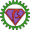BetaML.jl Documentation

Welcome to the documentation of the Beta Machine Learning toolkit.

The BetaML toolkit provides classical algorithms written in the Julia programming language useful to "learn" the relationship between some inputs and some outputs, with the objective to make accurate predictions of the output given new inputs ("supervised machine learning") or to better understand the structure of the data, perhaps hidden because of the high dimensionality ("unsupervised machine learning").

While specific packages exist for state-of-the art implementations of these algorithms (see the section "Alternative Packages"), thanks to the Just-In-Time compilation nature of Julia, BetaML is reasonably fast for datasets that fit in memory while keeping both the code and the usage as simple as possible.

Aside the algorithms themselves, BetaML provides many "utility" functions. Because algorithms are all self-contained in the library itself (you are invited to explore their source code by typing @edit functionOfInterest(par1,par2,...)), the utility functions have APIs that are coordinated with the algorithms, facilitating the "preparation" of the data for the analysis, the evaluation of the models or the implementation of several models in chains (pipelines). While BetaML doesn't provide itself tools for hyper-parameters optimisation or complex pipeline building tools, most models have an interface for the MLJ framework that allows it.

Aside Julia, BetaML can be accessed in R or Python using respectively JuliaCall and PyJulia. See the tutorial for details.

## Installation

The BetaML package is included in the standard Julia register, install it with:

• ] add BetaML

This package is split in several submodules, but all modules are re-exported at the root module level. This means that you can access their functionality by simply using BetaML.

using BetaML
myLayer = DenseLayer(2,3) # DenseLayer is defined in the Nn submodule
res     = kernelPerceptron([1.1 2.1; 5.3 4.2; 1.8 1.7], [-1,1,-1]) # kernelPerceptron is defined in the Perceptron module
@edit DenseLayer(2,3)     # Open a text editor with to the relevant source code

## Usage

New to BetaML or even to Julia / Machine Learning altogether? Start from the tutorial!

Detailed documentation for most algorithms can be retrieved using the inline Julia help system (just press the question mark ? and then, on the special help prompt help?>, type the function name) or on these pages under the section "Api (Reference Manual)" for the individual modules:

### MLJ interface

BetaML exports the following modules for usage with the MLJ toolkit:

• Perceptron models: PerceptronClassifier, KernelPerceptronClassifier, PegasosClassifier
• Decision trees/Random forest models: DecisionTreeClassifier, DecisionTreeRegressor, RandomForestClassifier, RandomForestRegressor
• Clustering models and derived models: KMeans, KMedoids, GMMClusterer, MissingImputator

Currently BetaML neural network models are not available in MLJ.

## Quick examples

(see the tutorial for a more step-by-step guide to the examples below and to other examples)

• Using an Artificial Neural Network for multinomial categorisation
# Load Modules
using BetaML, DelimitedFiles, Random, StatsPlots # Load the main module and ausiliary modules
Random.seed!(123); # Fix the random seed (to obtain reproducible results)

iris     = iris[shuffle(axes(iris, 1)), :] # Shuffle the records, as they aren't by default
x        = convert(Array{Float64,2}, iris[:,1:4])
y        = map(x->Dict("setosa" => 1, "versicolor" => 2, "virginica" =>3)[x],iris[:, 5]) # Convert the target column to numbers
y_oh     = oneHotEncoder(y) # Convert to One-hot representation (e.g. 2 => [0 1 0], 3 => [0 0 1])

# Split the data in training/testing sets
((xtrain,xtest),(ytrain,ytest),(ytrain_oh,ytest_oh)) = partition([x,y,y_oh],[0.8,0.2],shuffle=false)
(ntrain, ntest) = size.([xtrain,xtest],1)

# Define the Artificial Neural Network model
l1   = DenseLayer(4,10,f=relu) # Activation function is ReLU
l2   = DenseLayer(10,3)        # Activation function is identity by default
l3   = VectorFunctionLayer(3,3,f=softMax) # Add a (parameterless) layer whose activation function (softMax in this case) is defined to all its nodes at once
mynn = buildNetwork([l1,l2,l3],squaredCost,name="Multinomial logistic regression Model Sepal") # Build the NN and use the squared cost (aka MSE) as error function

# Training it (default to ADAM)
res = train!(mynn,scale(xtrain),ytrain_oh,epochs=100,batchSize=6) # Use optAlg=SGD (Stochastic Gradient Descent) by default

# Test it
ŷtrain        = predict(mynn,scale(xtrain))   # Note the scaling function
ŷtest         = predict(mynn,scale(xtest))
trainAccuracy = accuracy(ŷtrain,ytrain,tol=1) # 0.983
testAccuracy  = accuracy(ŷtest,ytest,tol=1)   # 1.0

# Visualise results
testSize    = size(ŷtest,1)
ŷtestChosen =  [argmax(ŷtest[i,:]) for i in 1:testSize]
groupedbar([ytest ŷtestChosen], label=["ytest" "ŷtest (est)"], title="True vs estimated categories") # All records correctly labelled !
plot(0:res.epochs,res.ϵ_epochs, ylabel="epochs",xlabel="error",legend=nothing,title="Avg. error per epoch on the Sepal dataset")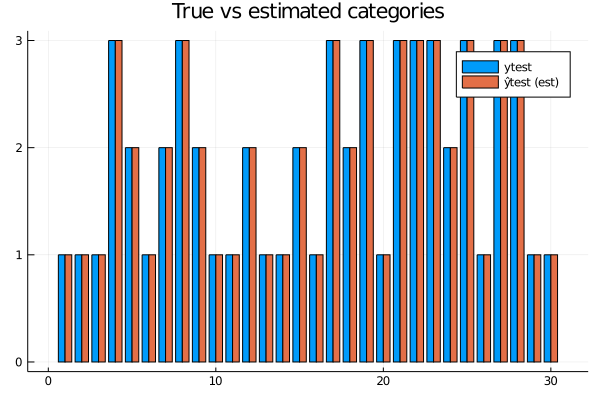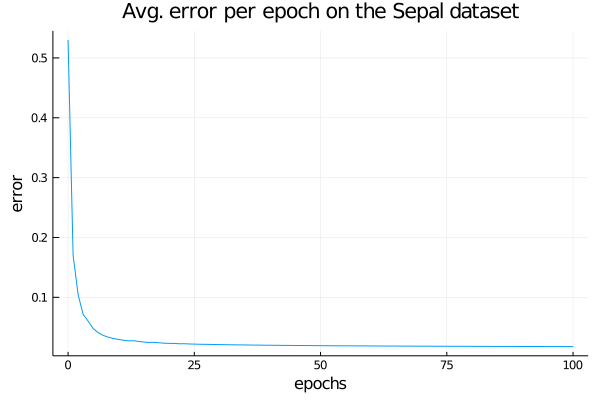• Using the Expectation-Maximisation algorithm for clustering
using BetaML, DelimitedFiles, Random, StatsPlots # Load the main module and ausiliary modules
Random.seed!(123); # Fix the random seed (to obtain reproducible results)

iris     = iris[shuffle(axes(iris, 1)), :] # Shuffle the records, as they aren't by default
x        = convert(Array{Float64,2}, iris[:,1:4])
x        = scale(x) # normalise all dimensions to (μ=0, σ=1)
y        = map(x->Dict("setosa" => 1, "versicolor" => 2, "virginica" =>3)[x],iris[:, 5]) # Convert the target column to numbers

# Get some ranges of minVariance and minCovariance to test
minVarRange   = collect(0.04:0.05:1.5)
minCovarRange = collect(0:0.05:1.45)

# Run the gmm(em) algorithm for the various cases...
sphOut  = [gmm(x,3,mixtures=[SphericalGaussian() for i in 1:3],minVariance=v, minCovariance=cv, verbosity=NONE) for v in minVarRange, cv in minCovarRange[1:1]]
diagOut  = [gmm(x,3,mixtures=[DiagonalGaussian() for i in 1:3],minVariance=v, minCovariance=cv, verbosity=NONE)  for v in minVarRange, cv in minCovarRange[1:1]]
fullOut = [gmm(x,3,mixtures=[FullGaussian() for i in 1:3],minVariance=v, minCovariance=cv, verbosity=NONE)  for v in minVarRange, cv in minCovarRange]

# Get the Bayesian information criterion (AIC is also available)
sphBIC = [sphOut[v,cv].BIC for v in 1:length(minVarRange), cv in 1:1]
diagBIC = [diagOut[v,cv].BIC for v in 1:length(minVarRange), cv in 1:1]
fullBIC = [fullOut[v,cv].BIC for v in 1:length(minVarRange), cv in 1:length(minCovarRange)]

# Compare the accuracy with true categories
sphAcc  = [accuracy(sphOut[v,cv].pₙₖ,y,ignoreLabels=true) for v in 1:length(minVarRange), cv in 1:1]
diagAcc = [accuracy(diagOut[v,cv].pₙₖ,y,ignoreLabels=true) for v in 1:length(minVarRange), cv in 1:1]
fullAcc = [accuracy(fullOut[v,cv].pₙₖ,y,ignoreLabels=true) for v in 1:length(minVarRange), cv in 1:length(minCovarRange)]

plot(minVarRange,[sphBIC diagBIC fullBIC[:,1] fullBIC[:,15] fullBIC[:,30]], markershape=:circle, label=["sph" "diag" "full (cov=0)" "full (cov=0.7)" "full (cov=1.45)"], title="BIC", xlabel="minVariance")
plot(minVarRange,[sphAcc diagAcc fullAcc[:,1] fullAcc[:,15] fullAcc[:,30]], markershape=:circle, label=["sph" "diag" "full (cov=0)" "full (cov=0.7)" "full (cov=1.45)"], title="Accuracies", xlabel="minVariance")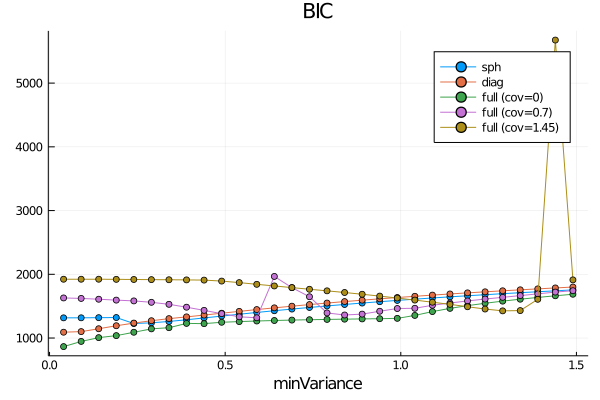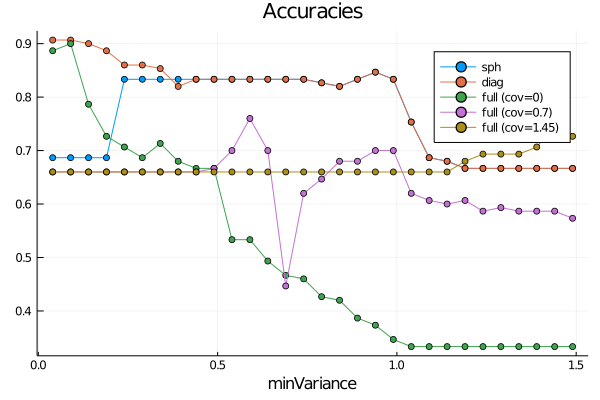• Further examples

Finally, you may want to give a look at the "test" folder. While the primary objective of the scripts under the "test" folder is to provide automatic testing of the BetaML toolkit, they can also be used to see how functions should be called, as virtually all functions provided by BetaML are tested there.

## Acknowledgements

The development of this package at the Bureau d'Economie Théorique et Appliquée (BETA, Nancy) was supported by the French National Research Agency through the Laboratory of Excellence ARBRE, a part of the “Investissements d'Avenir” Program (ANR 11 – LABX-0002-01).